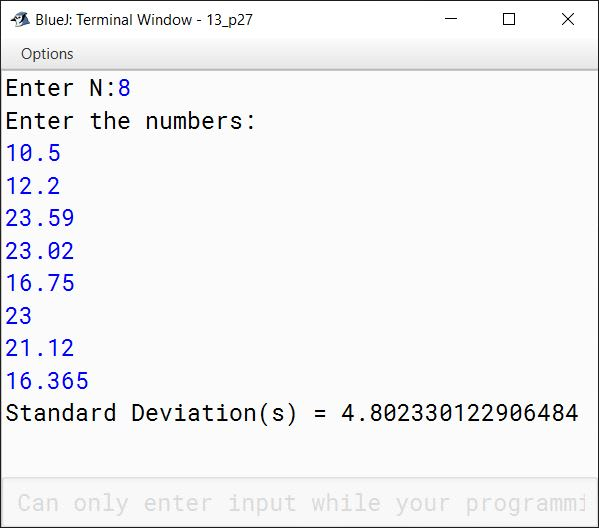# Arrays

## Multiple Choice Questions

#### Question 1

The size of an array that signifies the number of elements it can store is given using ........... brackets.

1. {}
2. [] ✓
3. ()
4. All of these

#### Question 2

Given array int x[] = {11, 22, 33, 44}; the value of x is ........... .

1. 11
2. 22 ✓
3. 33
4. Invalid value

#### Question 3

Given array int x[] = {11, 22, 33, 44}; the value of x[1+2] is ........... .

1. 11
2. 22
3. 33
4. 44 ✓

#### Question 4

If int arr[] = {3, 5, 7, 9}; what is the value of arr.length?

1. 3
2. 5
3. 4 ✓
4. Cannot be determined

#### Question 5

Given array int z[] = {15, 16, 17}; It will occupy ........... bytes in memory.

1. 3
2. 12 ✓
3. 24
4. 64

#### Question 6

A linear search ...........

1. can be used with sorted arrays only
2. can be used with unsorted arrays only
3. can be used with both sorted and unsorted arrays ✓
4. cannot be used with arrays

#### Question 7

A binary search

1. can be used with sorted arrays only ✓
2. can be used with unsorted arrays only
3. can be used with both sorted and unsorted arrays
4. cannot be used with arrays

#### Question 8

Which of the following statements is true?

1. Binary search is less efficient than the sequential search.
2. Binary search is less efficient than the linear search.
3. Binary search is more efficient than the sequential search. ✓
4. Binary search is as efficient as the sequential search.

#### Question 9

In ........... search, the algorithm uses the middle value of the array for the search operation.

1. Binary ✓
2. Linear
3. Bubble
4. Selection

#### Question 10

Which element is num of the array num?

1. 8th
2. 9th
3. 10th ✓
4. 11th

## Assignment Questions

#### Question 1

Write a program to initialise the given data in an array and find the minimum and maximum values along with the sum of the given elements.

Numbers: 2, 5, 4, 1, 3

Output:
Minimum value: 1
Maximum value: 5
Sum of the elements: 15

public class KboatMinMaxSum
{
public static void main(String args[]) {
int arr[] = {2, 5, 4, 1, 3};

int max = arr;
int min = arr;
int sum = 0;

for (int i = 0; i < arr.length; i++) {
if (arr[i] > max)
max = arr[i];

if (arr[i] < min)
min = arr[i];

sum += arr[i];
}

System.out.println("Minimum value: " + min);
System.out.println("Maximum value: " + max);
System.out.println("Sum of the elements: " + sum);
}
}
##### Output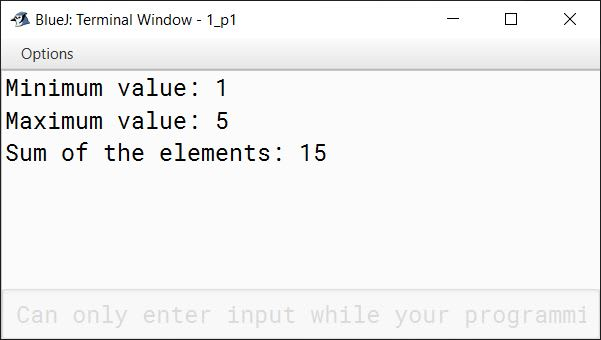#### Question 2

Differentiate between the following.

i. Array Declaration and Initialisation

Array declaration tells the compiler about the size and data type of the array so that the compiler can reserve the required memory for the array. This reserved memory is still empty. Array Initialisation assigns values to the array elements i.e. it stores values in the memory reserved for the array elements.

ii. int a and char a

int a is an array of int data type that can hold 10 integer values whereas char a is an array of char data type that can hold 10 characters.

iii. Sorting and Searching

SortingSearching
Sorting means to arrange the elements of the array in ascending or descending order.Searching means to search for a term or value in an array.
Bubble sort and Selection sort are examples of sorting techniques.Linear search and Binary search are examples of search techniques.

iv. Linear search and Binary search

Linear SearchBinary Search
Linear search works on sorted and unsorted arraysBinary search works on only sorted arrays (ascending or descending)
Each element of the array is checked against the target value until the element is found or end of the array is reachedArray is successively divided into 2 halves and the target element is searched either in the first half or in the second half
Linear Search is slowerBinary Search is faster

v. Selection sort and Bubble sort

Selection sortBubble sort
Selection Sort selects the smallest element from unsorted sub-array and swaps it with the leftmost unsorted element.Bubble Sort compares adjacent elements and swaps them if they are in wrong order.
Performs lesser number of swaps to sort the same array relative to Bubble SortPerforms more number of swaps to sort the array
Selection Sort is fasterBubble Sort is slower

#### Question 3

How does the linear search find an element in the array? Explain your answer with a suitable example.

In linear search, we start at the first element of the array and sequentially check each element of the list for the search value until a match is found or all the elements have been searched. As soon as the search value is found, the algorithm quits and returns the position (index) of the target value in the array.

For example, consider the following array:

int arr[] = {1, 8, 4, 7, 5};

We want to check if 7 is present in the array or not. Linear search will first check if 1 is equal to 7, then it will move on to the next element which is 8. It will keep doing this in a linear progression and when it reaches the element at index 3, it finds a match so it will give us this index 3 which means that 7 is present at index 3 of array arr.

#### Question 4

Explain the technique of Bubble Sort with an example.

Bubble Sort is a sorting algorithm that works by repeatedly iterating through the array, comparing each pair of adjoining elements and swapping them if they are in wrong order.

For example, consider the following unsorted array:

 9 5 2 3

Pass 1

First 9 is compared with 5 and as 9 is greater than 5 the elements are swapped:

 5 9 2 3

Next, 9 is compared with 2 and as 9 is greater than 2 the elements are swapped:

 5 2 9 3

Next, 9 is compared with 3 and as 9 is greater than 3 the elements are swapped:

 5 2 3 9

At the end of first pass, the highest element of the array is at the last position.

Pass 2

5 is compared with 2 and as 5 is greater than 2 the elements are swapped:

 2 5 3 9

Next, 5 is compared with 3 and as 5 is greater than 3 the elements are swapped:

 2 3 5 9

At the end of first pass, the second highest element of the array is in its correct position.

Pass 3

2 is compared with 3 and as 2 is less then 3 no swapping takes place.

 2 3 5 9

With this, the third and final pass ends and the elements of the array are in sorted order.

#### Question 5

Explain the technique of Selection Sort with an example.

Selection Sort divides the array into two parts — sorted sub-array and unsorted sub-array. In each pass, it finds the minimum element of the unsorted sub-array and swaps it with the leftmost unsorted element moving the sorted sub-array one element to the right.

For example, consider the following unsorted array:

 9 5 2 3

Pass 1

At the start, the smallest element of the array is selected (which is 2) and swapped with the element at 0th index (which is 9):

 2 5 9 3

At the end of the first pass, the smallest element is in its correct position. Length of sorted sub-array is 1 and unsorted sub-array is 3.

Pass 2

The smallest element of the unsorted sub-array is selected (which is 3) and swapped with the element at 1st index (which is 5):

 2 3 9 5

At the end of the second pass, length of sorted sub-array is 2 and unsorted sub-array is 2.

Pass 3

The smallest element of the unsorted sub-array is selected (which is 5) and swapped with the element at 2nd index (which is 9):

 2 3 5 9

With this, the third and final pass ends and the elements of the array are in sorted order.

#### Question 6

Why does Binary Search need a sorted array to perform the search operation?

In Binary Search, the array is repeatedly divided into two halves and the element is searched in that half whose last element is greater than or equal to the element being searched. For this reason, Binary Search needs a sorted array to perform the search operation.

#### Question 7

How does the binary search find the presence of an element quicker than the linear search?

As Binary Search repeatedly divides the array into two halves and performs the search in only one of those two halves, it needs to make fewer comparisons relative to Linear Search hence it is faster than Linear Search.

#### Question 8

Write a program to input integer elements into an array of size 20 and perform the following operations:

1. Display largest number from the array
2. Display smallest number from the array
3. Display sum of all the elements of the array

import java.util.Scanner;

public class KboatSDAMinMaxSum
{
public static void main(String args[]) {
Scanner in = new Scanner(System.in);
int arr[] = new int;
System.out.println("Enter 20 numbers:");
for (int i = 0; i < 20; i++) {
arr[i] = in.nextInt();
}
int min = arr, max = arr, sum = 0;
for (int i = 0; i < arr.length; i++) {
if (arr[i] < min)
min = arr[i];

if (arr[i] > max)
max = arr[i];

sum += arr[i];
}

System.out.println("Largest Number = " + max);
System.out.println("Smallest Number = " + min);
System.out.println("Sum = " + sum);
}
}
##### Output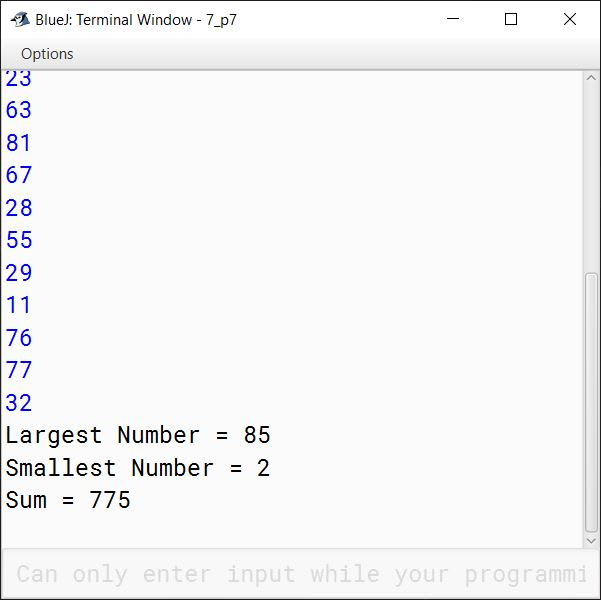#### Question 9

Declare and instantiate a one dimensional int array named evenNums with five elements. Use an initialiser list that contains the first five even integers, starting with 11.

int evenNums[] = {12, 14, 16, 18, 20};

#### Question 10

Suppose x is an array of type int[] with 50 elements. Write a code segment that will count and print the frequency of number 42 in the array.

int c = 0;
for (int i = 0; i < 50; i++) {
if (x[i] == 42) {
c++;
}
}
System.out.println("Frequency of 42 = " + c);

#### Question 11

A student wrote the following code segment, intending to print 11 22 33 44:

int arr[] = {11, 22, 33, 44};
for (int i = 1; i <= 4; i++)
System.out.println(arr[i]);

However, the program crashed with a run-time error. Can you explain the reason for this?

Array index starts at 0 not 1. In the given program, the for loop run from 1 to 4 whereas the indexes of the array range from 0 to 3. When i becomes 4, the program tries to access an index of arr that is not present in the array and this causes the program to crash. The correct way will be to run the for loop from 0 to 3 instead of 1 to 4.

#### Question 12

Write a code segment to compute the sum of all positive real numbers stored in the following array.

double numb[] = new double;

double sum = 0;
for (int i = 0; i < 50; i++) {
if (numb[i] > 0) {
sum += numb[i];
}
}
System.out.println("Sum of positive real numbers = " + sum);

#### Question 13

Write a code segment that finds the largest integer in this two-dimensional array.

int data[][] = new int;

int l = data;
for (int i = 0; i < 5; i++) {
for (int j = 0; j < 5; j++) {
if (data[i][j] > l) {
l = data[i][j];
}
}
}
System.out.println("Largest Element = " + l);

#### Question 14

Given the following declarations:

final int SIZE = 20;
char[] name = new char[SIZE];

i. Write an assignment statement that stores 'D' into the first element of the array name.

ii. Write an output statement that prints the value of the tenth element of the array name.

iii. Write a for statement that fills the array name with spaces.

i. name = 'D';

ii. System.out.println(name);

iii. For Statement:

for (int i = 0; i < SIZE; i++) {
name[i] = ' ';
}

#### Question 15

What happens in Java if you try to access an element that is outside the bounds of the array?

Accessing an element that is outside the bounds of the array results in a runtime error in the form of ArrayIndexOutOfBoundsException.

#### Question 16

Write Java statements for the following:

i. Create an array to hold 15 double values.

ii. Assign the value 10.5 to the last element in the array.

iii. Display the sum of the first and the last element.

iv. Write a loop that computes the sum of all elements in the array.

i. Create an array to hold 15 double values.

double arr[] = new double;

ii. Assign the value 10.5 to the last element in the array.

arr = 10.5;

iii. Display the sum of the first and the last element.

double r = arr + arr;
System.out.println("Result = " + r);

iv. Write a loop that computes the sum of all elements in the array.

double sum = 0;
for (int i = 0; i < 15; i++) {
sum += arr[i];
}
System.out.println("Sum = " + sum);

#### Question 17

Write a program to accept the year of graduation from school as an integer value from the user. Using the binary search technique on the sorted array of integers given below, output the message "Record exists" if the value input is located in the array. If not, output the message "Record does not exist".
Sample Input:

nnnnnnnnnn
1982198719931996199920032006200720092010

import java.util.Scanner;

{
public static void main(String args[]) {
Scanner in = new Scanner(System.in);
int n[] = {1982, 1987, 1993, 1996, 1999, 2003, 2006, 2007, 2009, 2010};

System.out.print("Enter graduation year to search: ");
int year = in.nextInt();

int l = 0, h = n.length - 1, idx = -1;
while (l <= h) {
int m = (l + h) / 2;
if (n[m] == year) {
idx = m;
break;
}
else if (n[m] < year) {
l = m + 1;
}
else {
h = m - 1;
}
}

if (idx == -1)
System.out.println("Record does not exist");
else
System.out.println("Record exists");
}
}
##### Output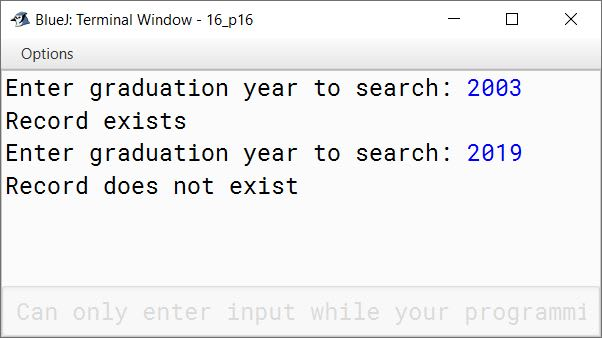#### Question 18

Write a program that reads ten integers and displays them in the reverse order in which they were read.

import java.util.Scanner;

public class KboatSDAReverse
{
public static void main(String args[]) {

Scanner in = new Scanner(System.in);
int arr[] = new int;

System.out.println("Enter 10 integers:");
for (int i = 0; i < 10; i++) {
arr[i] = in.nextInt();
}

System.out.println("Integers in reverse order:");
for (int i = 9; i >= 0; i--) {
System.out.print(arr[i] + " ");
}
}
}
##### Output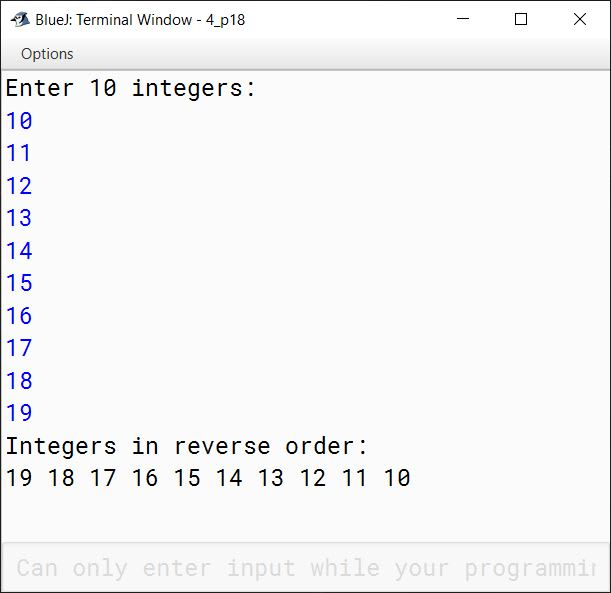#### Question 19

Write a program that reads a long number, counts and displays the occurrences of each digit in it.

import java.util.Scanner;

public class KboatSDANumber
{
public static void main(String args[]) {

Scanner in = new Scanner(System.in);
System.out.print("Enter a number: ");
long num = in.nextLong();
int dCount[] = new int;

while (num != 0) {
int d = (int)(num % 10);
dCount[d] = dCount[d] + 1;
num /= 10;
}

System.out.println("Digit\tOccurence");
for (int i = 0; i < 10; i++) {
if (dCount[i] != 0) {
System.out.println(i + "\t" + dCount[i]);
}
}
}
}
##### Output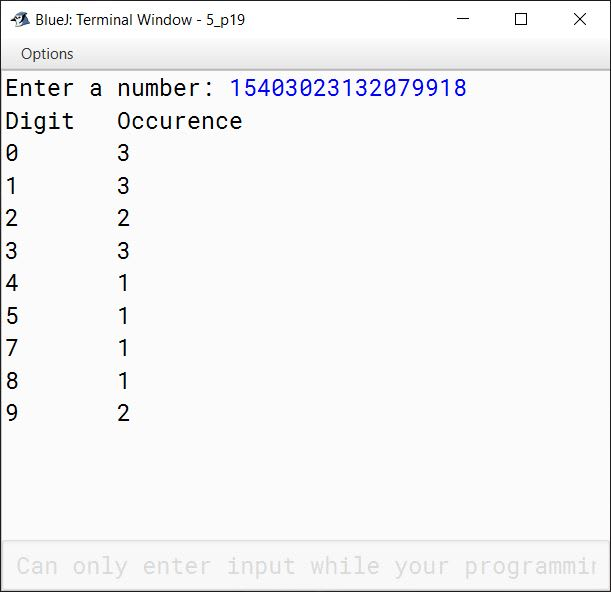#### Question 20

Write a program to input 10 integer elements in an array and sort them in descending order using bubble sort technique.

import java.util.Scanner;

public class KboatBubbleSortDsc
{
public static void main(String args[]) {
Scanner in = new Scanner(System.in);
int n = 10;
int arr[] = new int[n];

System.out.println("Enter the elements of the array:");
for (int i = 0; i < n; i++) {
arr[i] = in.nextInt();
}

//Bubble Sort
for (int i = 0; i < n - 1; i++) {
for (int j = 0; j < n - i - 1; j++) {
if (arr[j] < arr[j + 1]) {
int t = arr[j];
arr[j] = arr[j+1];
arr[j+1] = t;
}
}
}

System.out.println("Sorted Array:");
for (int i = 0; i < n; i++) {
System.out.print(arr[i] + " ");
}
}
}
##### Output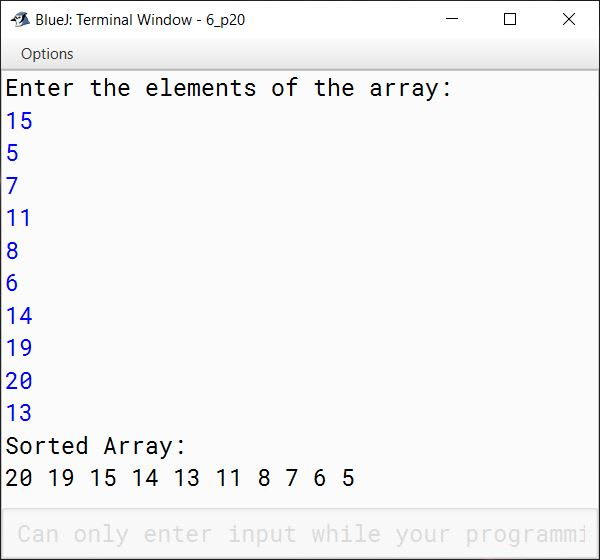#### Question 21

Write a program to perform binary search on a list of integers given below, to search for an element input by the user. If it is found display the element along with its position, otherwise display the message "Search element not found".

5, 7, 9, 11, 15, 20, 30, 45, 89, 97

import java.util.Scanner;

public class KboatBinarySearch
{
public static void main(String args[]) {

Scanner in = new Scanner(System.in);
int arr[] = {5, 7, 9, 11, 15, 20, 30, 45, 89, 97};

System.out.print("Enter number to search: ");
int n = in.nextInt();

int l = 0, h = arr.length - 1, index = -1;
while (l <= h) {
int m = (l + h) / 2;
if (arr[m] < n)
l = m + 1;
else if (arr[m] > n)
h = m - 1;
else {
index = m;
break;
}

}

if (index == -1) {
}
else {
System.out.println(n + " found at position " + index);
}
}
}
##### Output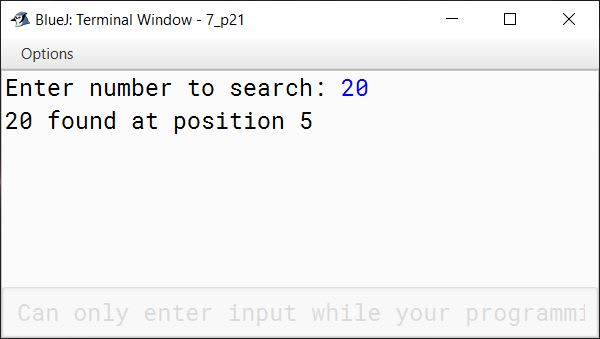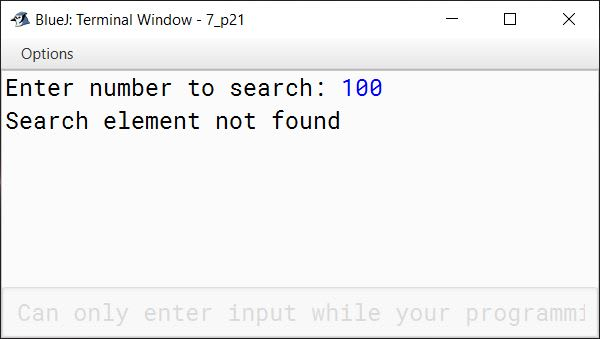#### Question 22

Write a program to store 6 elements in an array P and 4 elements in an array Q. Now, produce a third array R, containing all the elements of array P and Q. Display the resultant array.

InputInputOutput
P[ ]Q[ ]R[ ]
4194
6236
171
282
3 3
10 10
19
23
7
8

import java.util.Scanner;

public class Kboat3Arrays
{
public static void main(String args[]) {

Scanner in = new Scanner(System.in);

int P[] = new int;
int Q[] = new int;
int R[] = new int;
int i = 0;

System.out.println("Enter 6 elements of array P:");
for (i = 0; i < P.length; i++) {
P[i] = in.nextInt();
}

System.out.println("Enter 4 elements of array Q:");
for (i = 0; i < Q.length; i++) {
Q[i] = in.nextInt();
}

i = 0;
while(i < P.length) {
R[i] = P[i];
i++;
}

int j = 0;
while(j < Q.length) {
R[i++] = Q[j++];
}

System.out.println("Elements of Array R:");
for (i = 0; i < R.length; i++) {
System.out.print(R[i] + " ");
}
}
}
##### Output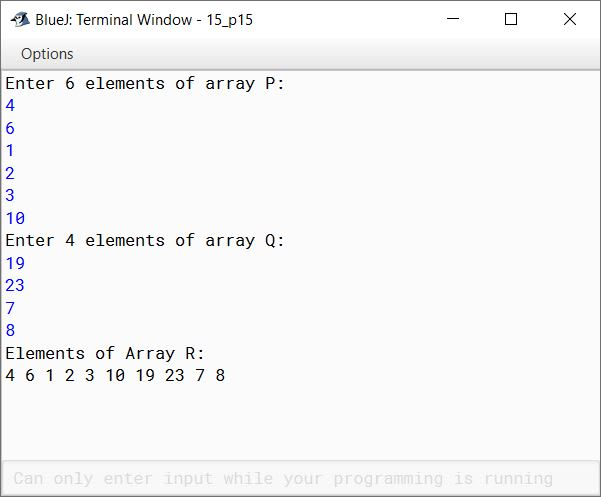#### Question 23

The annual examination result of 50 students in a class is tabulated in a Single Dimensional Array (SDA) as follows:

Roll No.Subject ASubject BSubject C
............................
............................
............................

Write a program to read the data, calculate and display the following:
(a) Average marks obtained by each student.
(b) Print the roll number and the average marks of the students whose average is above. 80.
(c) Print the roll number and the average marks of the students whose average is below 40.

import java.util.Scanner;

public class KboatExamResult
{
public static void main(String args[]) {
final int TOTAL_STUDENTS = 50;
Scanner in = new Scanner(System.in);

int rollNo[] = new int[TOTAL_STUDENTS];
int sA[] = new int[TOTAL_STUDENTS];
int sB[] = new int[TOTAL_STUDENTS];
int sC[] = new int[TOTAL_STUDENTS];
double avg[] = new double[TOTAL_STUDENTS];

for (int i = 0; i < TOTAL_STUDENTS; i++) {
System.out.println("Enter student " + (i+1) + " details:");
System.out.print("Roll No: ");
rollNo[i] = in.nextInt();
System.out.print("Subject A Marks: ");
sA[i] = in.nextInt();
System.out.print("Subject B Marks: ");
sB[i] = in.nextInt();
System.out.print("Subject C Marks: ");
sC[i] = in.nextInt();
avg[i] = (sA[i] + sB[i] + sC[i]) / 3.0;
}

System.out.println("\nRoll No\tAverage Marks");
for (int i = 0; i < TOTAL_STUDENTS; i++) {
System.out.println(rollNo[i] + "\t" + avg[i]);
}

System.out.println("\nStudents with Average above 80:");
for (int i = 0; i < TOTAL_STUDENTS; i++) {
if (avg[i] > 80)
System.out.println(rollNo[i] + "\t" + avg[i]);
}

System.out.println("\nStudents with Average below 40:");
for (int i = 0; i < TOTAL_STUDENTS; i++) {
if (avg[i] < 40)
System.out.println(rollNo[i] + "\t" + avg[i]);
}
}
}
##### Output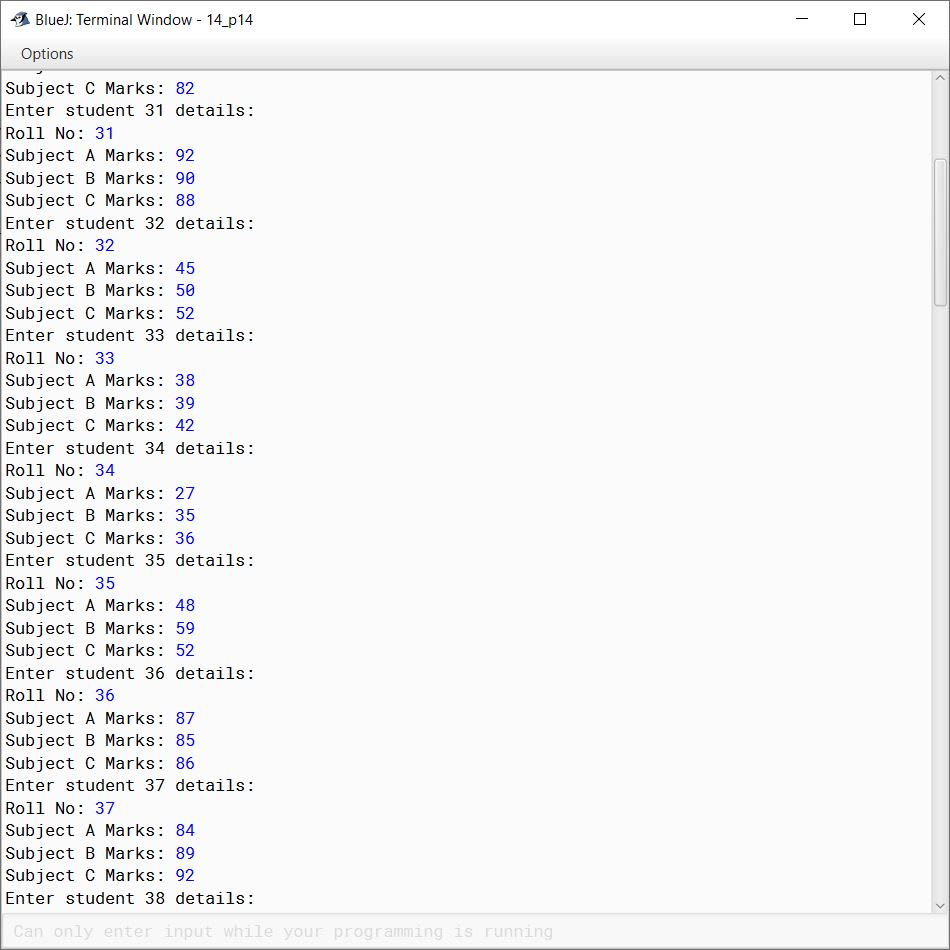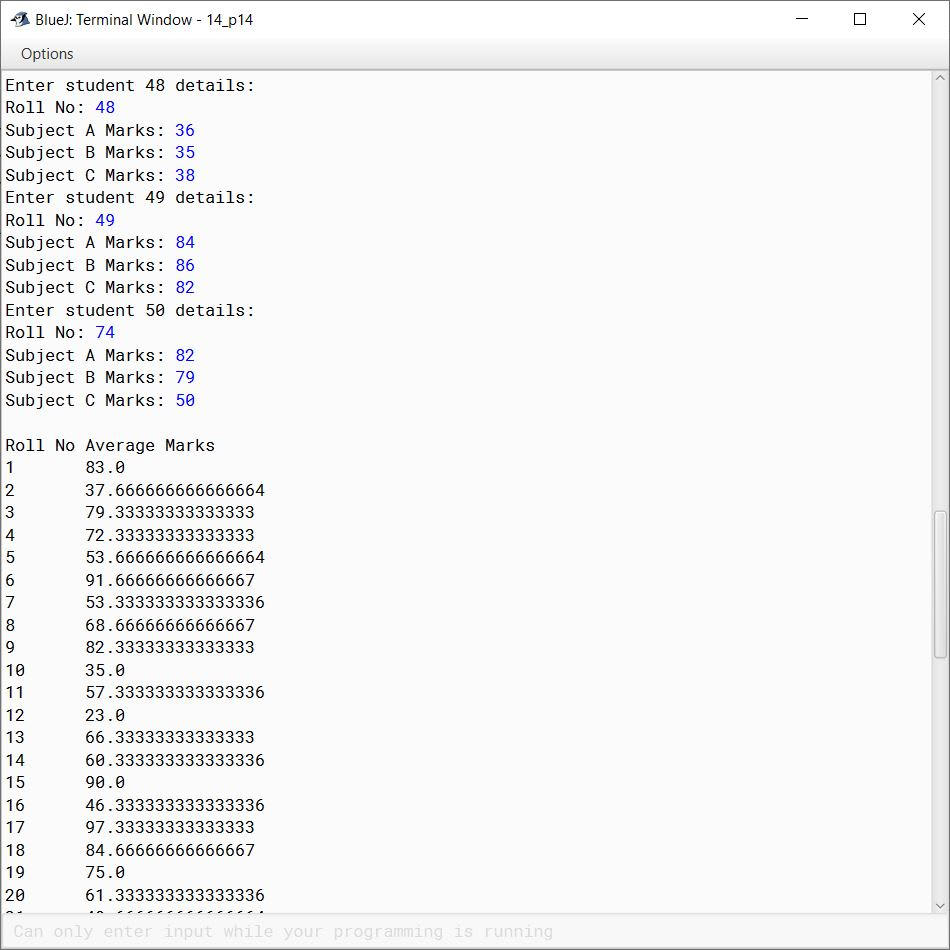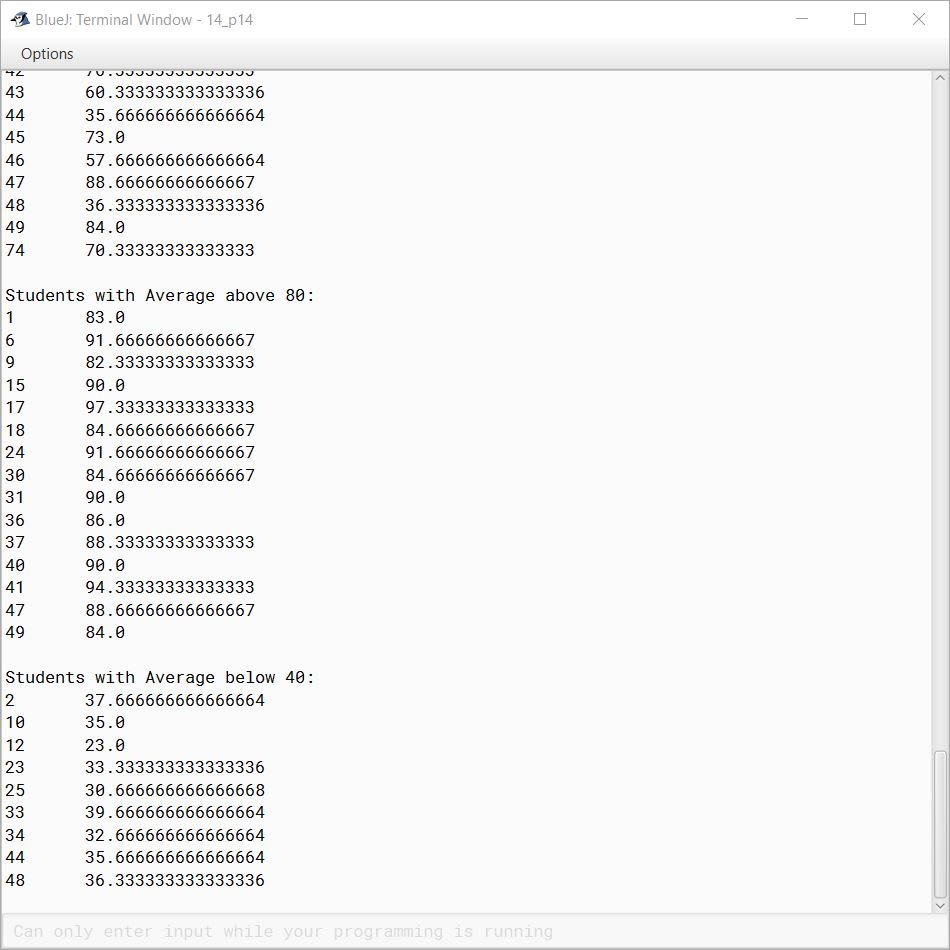#### Question 24

Declare a single dimensional array of size 28 to store daily temperatures for the month of February. Using this structure, write a program to find:

1. The hottest day of the month
2. The coldest day of the month
3. The average temperature of the month

import java.util.Scanner;

public class KboatFebTemp
{
public static void main(String args[]) {
Scanner in = new Scanner(System.in);
double febTemp[] = new double;
int n = febTemp.length;

System.out.println("Enter Feb daily temperatures:");
for (int i = 0; i < n; i++) {
febTemp[i] = in.nextDouble();
}

double sum = 0.0;
int low = 0, high = 0;
for (int i = 0; i < n; i++) {
if (febTemp[i] < febTemp[low])
low = i;

if (febTemp[i] > febTemp[high])
high = i;

sum += febTemp[i];
}

double avg = sum / n;

System.out.println("Hottest day = " + (high + 1));
System.out.println("Coldest day = " + (low + 1));
System.out.println("Average Temperature = " + avg);
}
}
##### Output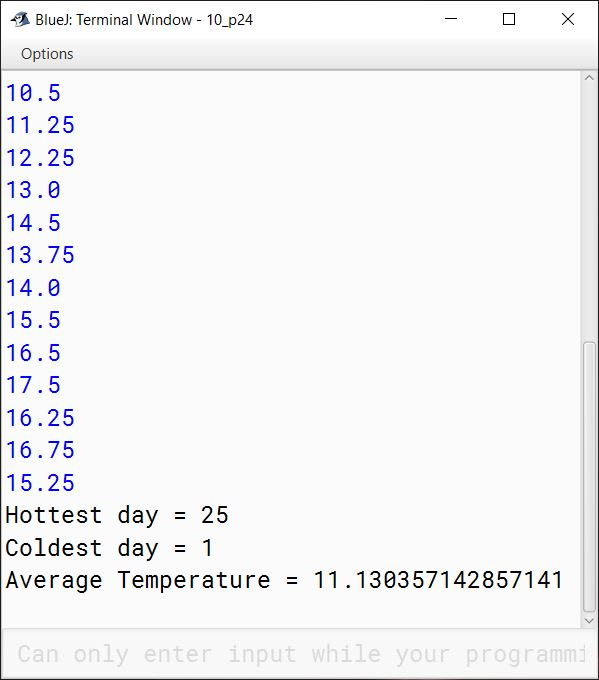#### Question 25

Repeat the above exercise using a double two-dimensional array with 4 rows and 7 columns to store daily temperatures for the month of February.

import java.util.Scanner;

public class KboatDDAFebTemp
{
public static void main(String args[]) {
Scanner in = new Scanner(System.in);
double febTemp[][] = new double;

System.out.println("Enter Feb daily temperatures:");
for (int i = 0; i < 4; i++) {
for (int j = 0; j < 7; j++) {
febTemp[i][j] = in.nextDouble();
}
}

double sum = 0.0;
int lx = 0, ly = 0, hx = 0, hy = 0;
for (int i = 0; i < 4; i++) {
for (int j = 0; j < 7; j++) {
if (febTemp[i][j] < febTemp[lx][ly]) {
lx = i;
ly = j;
}

if (febTemp[i][j] > febTemp[hx][hy]) {
hx = i;
hy = j;
}

sum += febTemp[i][j];

}
}

double avg = sum / 28;

System.out.println("Hottest day = " + (hx * 7 + hy + 1));
System.out.println("Coldest day = " + (lx * 7 + ly + 1));
System.out.println("Average Temperature = " + avg);
}
}
##### Output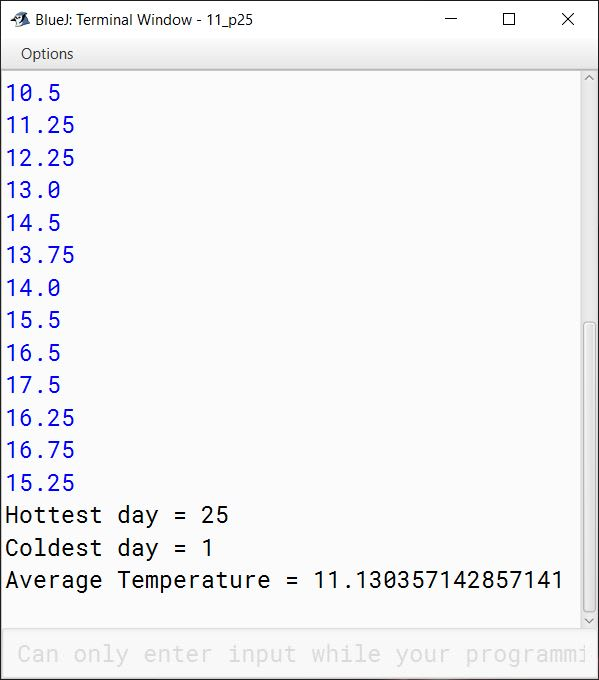#### Question 26

The weekly hours of all employees of ABC Consulting Ltd. are stored in a two-dimensional array. Each row records an employee's 7-day work hours with seven columns. For example, the following array stores the work hours of five employees. Write a program that displays employees and their total hours in decreasing order of the total hours.

S. No.MonTueWedThuFriSatSun
Employee 085111100
Employee 111602224
Employee 245410431
Employee 347312620
Employee 43832440

import java.util.Scanner;

public class KboatABCConsulting
{
public static void main(String args[]) {

Scanner in = new Scanner(System.in);
System.out.print("Enter total number of employees: ");
int numEmps = in.nextInt();

int workHours[][] = new int[numEmps];
int empArr[] = new int[numEmps];
int hours[] = new int[numEmps];

//Enter employee hours in 2D array
for (int i = 0; i < numEmps; i++) {
System.out.println("Enter hours for Employee " + i);
for (int j = 0; j < 7; j++) {
workHours[i][j] = in.nextInt();
}
}

//Calculate total hours for each employee
for (int i = 0; i < numEmps; i++) {
int totalHours = 0;
for (int j = 0; j < 7; j++) {
totalHours += workHours[i][j];
}
empArr[i] = i;
hours[i] = totalHours;
}

//Sort total hours hours in decreasing order
for (int i = 0; i < numEmps - 1; i++) {
int index = i;
for (int j = i + 1; j < numEmps; j++) {
if (hours[j] > hours[index])
index = j;
}
int t = hours[i];
hours[i] = hours[index];
hours[index] = t;

t = empArr[i];
empArr[i] = empArr[index];
empArr[index] = t;
}

//Display sorted total hours
for (int i = 0; i < numEmps; i++)
System.out.println("Employee " + empArr[i]
+ "\t" + hours[i]);
}
}
##### Output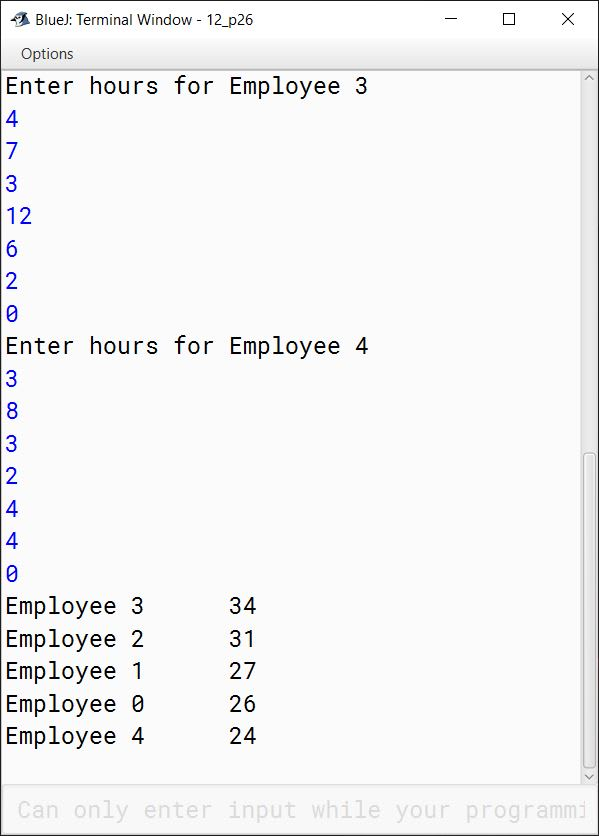#### Question 27

Write a program that computes the standard deviation of N real numbers. The standard deviation s is computed according to:

$s=\sqrt{\dfrac{(x_1 - \overset{-}{x})^2 + (x_2 - \overset{-}{x})^2 + ... + (x_N - \overset{-}{x})^2}{N}}$

The variable $\overset{-}{x}$ is the average of N input values $x_1$ through $x_N$. The program first prompts the user for N and then declares an array of size N.

import java.util.Scanner;

public class KboatStdDev
{
public static void main(String args[]) {
Scanner in = new Scanner(System.in);

System.out.print("Enter N:");
int n = in.nextInt();

double a[] = new double[n];

System.out.println("Enter the numbers:");
for (int i = 0; i < n; i++) {
a[i] = in.nextDouble();
}

double sum = 0;
for (int i = 0; i < n; i++) {
sum += a[i];
}

double avg = sum / n;
double z = 0;
for (int i = 0; i < n; i++) {
z += Math.pow(a[i] - avg, 2);
}

double s = Math.sqrt(z / n);

System.out.println("Standard Deviation(s) = " + s);
}
}
##### Output